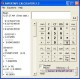Quadratic! 1.0 Quadratic! widget solves almost any quadratic equation. FreewareQuadratic Formula Helper 0.1 Quadratic Formula Helper is a small application for quickly finding the value(s) of X from a quadratic formula. FreewareQuadratic X 2.1 Quadratic is a simple program that calculates the answers to the quadratic formula. FreewareQuadratic Formula 1.1 Quadratic Formula is a simple program that will solve the Quadratic Formula for you. FreewareQuadEquations 2.0.1 QuadEquations facilitates the teaching and learning of quadratic equations. SharewareSolve 1.0.1 Solve is a simple application that calculates the zeros (x-intercepts) of any arbitrary quadratic equation. FreewarePapertape Calculator 3.1 A full function calculator that records all entries to a paper tape. Graphics SharewareVvidget 10.4.37 Vvidget is the premier chart and graph system for Mac OS X. FreewareCalcPad X 1.1 CalcPad X is a "word processor" and evaluator for mathematical expressions! CalcPad X lets you type an expression and evaluate it with a single click. SharewareAlgeWorksheets 2.0 AlgeWorksheets - Generate and print pre-algebra and algebra worksheets or test papers in minutes. Shareware